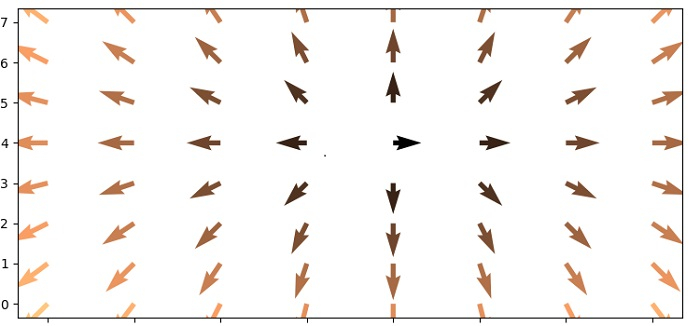# Plot a vector field over the axes in Python Matplotlib?

To plot a vector field over the axes in matplotlib, we can take the following steps −

• Set the figure size and adjust the padding between and around the subplots.

• Make X, Y, T, R, U and V data points using numpy.

• Add an axes to the current figure and make it the current axes.

• Plot a 3D field of arrows using quiver() method.

• To display the figure, use show() method.

## Example

import numpy as np
import matplotlib.pyplot as plt

plt.rcParams["figure.figsize"] = [7.50, 3.50]
plt.rcParams["figure.autolayout"] = True

n = 8
X, Y = np.mgrid[0:n, 0:n]
T = np.arctan2(Y - n / 2., X - n/2.)
R = 10 + np.sqrt((Y - n / 2.0) ** 2 + (X - n / 2.0) ** 2)
U, V = R * np.cos(T), R * np.sin(T)

plt.axes([0.025, 0.025, 0.95, 0.95])
plt.quiver(X, Y, U, V, R, cmap="copper")

plt.show()

## Output